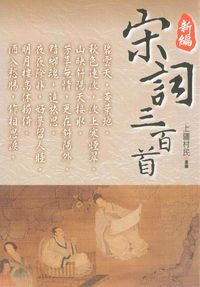### 新編宋詞三百首

• 點閱：1
• 其他題名：宋詞三百首 新編宋詞300首
• 作者：
• 出版年：2015[民104]
• 出版社：新潮社出版 聯合發行代理
• 出版地：新北市
• 集叢名：當代文庫:27
• ISBN：978-986-316-616-0 ; 986-316-616-2

##簡介

《宋詞三百首》是1924年編定輯錄的宋詞選。

##章節

• 宴山亭　趙佶(p.33)
• 木蘭花　錢惟演(p.34)
• 蘇幕遮　范仲淹(p.34)
• 御街行　范仲淹(p.35)
• 千秋歲　張先(p.36)
• 菩薩蠻　張先(p.37)
• 醉垂鞭　張先(p.38)
• 一叢花　張先(p.39)
• 天仙子　張先(p.40)
• 青門引　張先(p.41)
• 浣溪沙　晏殊(p.42)
• 浣溪沙　晏殊(p.42)
• 清平樂　晏殊(p.43)
• 清平樂　晏殊(p.44)
• 木蘭花　晏殊(p.44)
• 木蘭花　晏殊(p.45)
• 木蘭花　晏殊(p.46)
• 踏莎行　晏殊(p.47)
• 踏莎行　晏殊(p.48)
• 蝶戀花　晏殊(p.49)
• 鳳簫吟　韓縝(p.50)
• 木蘭花　宋祁(p.51)
• 采桑子　歐陽修(p.52)
• 訴衷情　歐陽修(p.53)
• 踏莎行　歐陽修(p.54)
• 蝶戀花　歐陽修(p.55)
• 蝶戀花　歐陽修(p.56)
• 蝶戀花　歐陽修(p.56)
• 木蘭花　歐陽修(p.57)
• 浪淘沙　歐陽修(p.58)
• 青玉案　歐陽修(p.59)
• 曲玉管　柳永(p.60)
• 雨霖鈴　柳永(p.61)
• 蝶戀花　柳永(p.62)
• 采蓮令　柳永(p.63)
• 浪淘沙慢　柳永(p.64)
• 定風波　柳永(p.66)
• 少年游　柳永(p.67)
• 戚氏　柳永(p.68)
• 夜半樂　柳永(p.69)
• 玉胡蝶　柳永(p.70)
• 八聲甘州　柳永(p.71)
• 迷神引　柳永(p.73)
• 竹馬子　柳永(p.74)
• 桂枝香　王安石(p.75)
• 千秋歲引　王安石(p.76)
• 清平樂　王安國(p.77)
• 臨江仙　晏幾道(p.78)
• 蝶戀花　晏幾道(p.79)
• 蝶戀花　晏幾道(p.80)
• 鷓鴣天　晏幾道(p.81)
• 生查子　晏幾道(p.82)
• 木蘭花　晏幾道(p.82)
• 木蘭花　晏幾道(p.83)
• 清平樂　晏幾道(p.84)
• 阮郎歸　晏幾道(p.85)
• 阮郎歸　晏幾道(p.86)
• 六么令　晏幾道(p.86)
• 御街行　晏幾道(p.88)
• 虞美人　晏幾道(p.88)
• 留春令　晏幾道(p.89)
• 思遠人　晏幾道(p.90)
• 水調歌頭　蘇軾(p.91)
• 水龍吟　蘇軾(p.92)
• 永遇樂　蘇軾(p.93)
• 洞仙歌　蘇軾(p.94)
• 卜算子　蘇軾(p.95)
• 青玉案　蘇軾(p.96)
• 臨江仙　蘇軾(p.97)
• 定風波　蘇軾(p.97)
• 江城子　蘇軾(p.98)
• 賀新郎　蘇軾(p.99)
• 望海潮　秦觀(p.100)
• 八六子　秦觀(p.101)
• 滿庭芳　秦觀(p.102)
• 滿庭芳　秦觀(p.103)
• 減字木蘭花　秦觀(p.104)
• 浣溪沙　秦觀(p.104)
• 阮郎歸　秦觀(p.105)
• 綠頭鴨　晁元禮(p.106)
• 蝶戀花　趙令畤(p.107)
• 蝶戀花　趙令畤(p.107)
• 清平樂　趙令畤(p.108)
• 水龍吟　晁補之(p.109)
• 憶少年　晁補之(p.110)
• 洞仙歌　晁補之(p.110)
• 臨江仙　晁沖之(p.111)
• 虞美人　舒亶(p.112)
• 漁家傲　朱服(p.113)
• 惜分飛　毛滂(p.113)
• 菩薩蠻　陳克(p.114)
• 菩薩蠻　陳克(p.115)
• 洞仙歌　李元膺(p.116)
• 青門飲　時彥(p.117)
• 謝池春　李之儀(p.118)
• 卜算子　李之儀(p.118)
• 瑞龍吟　周邦彥(p.119)
• 風流子　周邦彥(p.120)
• 蘭陵王　周邦彥(p.121)
• 瑣窗寒　周邦彥(p.122)
• 六醜　周邦彥(p.123)
• 夜飛鵲　周邦彥(p.125)
• 滿庭芳　周邦彥(p.126)
• 過秦樓　周邦彥(p.127)
• 花犯　周邦彥(p.128)
• 大酺　周邦彥(p.129)
• 解語花　周邦彥(p.130)
• 蝶戀花　周邦彥(p.131)
• 解連環　周邦彥(p.132)
• 拜星月慢　周邦彥(p.132)
• 關河令　周邦彥(p.133)
• 綺寮怨　周邦彥(p.134)
• 尉遲杯　周邦彥(p.135)
• 西河　周邦彥(p.135)
• 瑞鶴仙　周邦彥(p.136)
• 浪淘沙慢　周邦彥(p.137)
• 應天長　周邦彥(p.138)
• 夜游宮　周邦彥(p.139)
• 青玉案　賀鑄(p.139)
• 感皇恩　賀鑄(p.140)
• 薄倖　賀鑄(p.141)
• 浣溪沙　賀鑄(p.142)
• 浣溪沙　賀鑄(p.142)
• 石州慢　賀鑄(p.143)
• 蝶戀花　賀鑄(p.144)
• 天門謠　賀鑄(p.145)
• 天香　賀鑄(p.145)
• 望湘人　賀鑄(p.147)
• 綠頭鴨　賀鑄(p.148)
• 石州慢　張元幹(p.149)
• 蘭陵王　張元幹(p.149)
• 賀新郎　葉夢得(p.151)
• 虞美人　葉夢得(p.152)
• 點絳脣　汪藻(p.153)
• 喜遷鶯　劉一止(p.153)
• 高陽臺　韓疁(p.154)
• 漢宮春　李邴(p.155)
• 臨江仙　陳與義(p.156)
• 臨江仙　陳與義(p.156)
• 蘇武慢　蔡伸(p.157)
• 柳梢青　蔡伸(p.158)
• 鷓鴣天　周紫芝(p.158)
• 踏莎行　周紫芝(p.159)
• 帝臺春　李甲(p.160)
• 三臺　万俟詠(p.161)
• 憶王孫　李重元(p.161)
• 二郎神　徐伸(p.163)
• 江神子慢　田為(p.164)
• 驀山溪　曹組(p.165)
• 賀新郎　李玉(p.165)
• 燭影搖紅　廖世美(p.167)
• 薄倖　呂濱老(p.168)
• 南浦　魯逸仲(p.169)
• 滿江紅　岳飛(p.169)
• 燭影搖紅　張掄(p.170)
• 水龍吟　程垓(p.171)
• 六州歌頭　張孝祥(p.172)
• 念奴嬌　張孝祥(p.173)
• 六州歌頭　韓元吉(p.174)
• 好事近　韓元吉(p.175)
• 瑞鶴仙　袁去華(p.176)
• 劍器近　袁去華(p.176)
• 安公子　袁去華(p.177)
• 瑞鶴仙　陸淞(p.178)
• 卜算子　陸游(p.179)
• 水龍吟　陳亮(p.179)
• 憶秦娥　范成大(p.180)
• 眼兒媚　范成大(p.181)
• 霜天曉角　范成大(p.182)
• 賀新郎　辛棄疾(p.182)
• 念奴嬌　辛棄疾(p.183)
• 漢宮春　辛棄疾(p.184)
• 賀新郎　辛棄疾(p.185)
• 水龍吟　辛棄疾(p.186)
• 摸魚兒　辛棄疾(p.187)
• 永遇樂　辛棄疾(p.188)
• 木蘭花慢　辛棄疾(p.189)
• 祝英臺近　辛棄疾(p.189)
• 青玉案　辛棄疾(p.190)
• 鷓鴣天　辛棄疾(p.191)
• 菩薩蠻　辛棄疾(p.192)
• 點緯脣　姜夔(p.193)
• 鷓鴣天　姜夔(p.193)
• 踏莎行　姜夔(p.194)
• 慶宮春　姜夔(p.195)
• 齊天樂　姜夔(p.196)
• 琵琶仙　姜夔(p.197)
• 八歸　姜夔(p.198)
• 念奴嬌　姜夔(p.199)
• 揚州慢　姜夔(p.201)
• 長亭怨慢　姜夔(p.202)
• 淡黃柳　姜夔(p.203)
• 暗香　姜夔(p.204)
• 疏影　姜夔(p.205)
• 翠樓吟　姜夔(p.206)
• 杏花天　姜夔(p.207)
• 一萼紅　姜夔(p.208)
• 霓裳中序第一　姜夔(p.209)
• 小重山　章良能(p.210)
• 唐多令　劉過(p.210)
• 木蘭花　嚴仁(p.211)
• 風入松　俞國寶(p.212)
• 滿庭芳　張鎡(p.213)
• 宴山亭　張鎡(p.214)
• 綺羅香　史達祖(p.215)
• 雙雙燕　史達祖(p.216)
• 東風第一枝　史達祖(p.217)
• 喜遷鶯　史達祖(p.217)
• 三姝媚　史達祖(p.218)
• 秋霽　史達祖(p.219)
• 夜合花　史達祖(p.220)
• 玉胡蝶　史達祖(p.221)
• 八歸　史達祖(p.222)
• 生查子　劉克莊(p.223)
• 賀新郎　劉克莊(p.223)
• 賀新郎　劉克莊(p.224)
• 木蘭花　劉克莊(p.225)
• 江城子　盧祖皋(p.226)
• 宴清都　盧祖皋(p.227)
• 南鄉子　潘牥(p.228)
• 瑞鶴仙　陸叡(p.229)
• 渡江雲　吳文英(p.229)
• 夜合花　吳文英(p.230)
• 霜葉飛　吳文英(p.231)
• 宴清都　吳文英(p.232)
• 齊天樂　吳文英(p.234)
• 花犯　吳文英(p.235)
• 浣溪沙　吳文英(p.236)
• 浣溪沙　吳文英(p.237)
• 點緯脣　吳文英(p.237)
• 祝英臺近　吳文英(p.238)
• 祝英臺近　吳文英(p.238)
• 澡蘭香　吳文英(p.239)
• 風入松　吳文英(p.240)
• 鶯啼序　吳文英(p.241)
• 惜黃花慢　吳文英(p.243)
• 高陽臺　吳文英(p.244)
• 高陽臺　吳文英(p.245)
• 三姝媚　吳文英(p.246)
• 八聲甘州　吳文英(p.248)
• 踏莎行　吳文英(p.248)
• 瑞鶴仙　吳文英(p.249)
• 鷓鴣天　吳文英(p.250)
• 夜游宮　吳文英(p.251)
• 賀新郎　吳文英(p.251)
• 唐多令　吳文英(p.252)
• 湘春夜月　黃孝邁(p.253)
• 大有　潘希白(p.254)
• 青玉案　黃公紹(p.255)
• 摸魚兒　朱嗣發(p.255)
• 蘭陵王　劉辰翁(p.256)
• 寶鼎現　劉辰翁(p.257)
• 永遇樂　劉辰翁(p.258)
• 摸魚兒　劉辰翁(p.259)
• 高陽臺　周密(p.260)
• 瑤華　周密(p.261)
• 玉京秋　周密(p.262)
• 曲游春　周密(p.263)
• 花犯　周密(p.264)
• 瑞鶴仙　蔣捷(p.265)
• 賀新郎　蔣捷(p.266)
• 女冠子　蔣捷(p.267)
• 高陽臺　張炎(p.268)
• 渡江雲　張炎(p.269)
• 八聲甘州　張炎(p.270)
• 解連環　張炎(p.271)
• 疏影　張炎(p.272)
• 月下笛　張炎(p.272)
• 天香　王沂孫(p.273)
• 眉嫵　王沂孫(p.274)
• 齊天樂　王沂孫(p.275)
• 長亭怨慢　王沂孫(p.276)
• 高陽臺　王沂孫(p.277)
• 法曲獻仙音　王沂孫(p.278)
• 疏影　彭元遜(p.279)
• 六醜　彭元遜(p.280)
• 紫萸香慢　姚雲文(p.281)
• 金明池　僧揮(p.282)
• 鳳凰臺上憶吹簫　李清照(p.283)
• 醉花陰　李清照(p.284)
• 聲聲慢　李清照(p.284)
• 念奴嬌　李清照(p.285)
• 永遇樂　李清照(p.286)